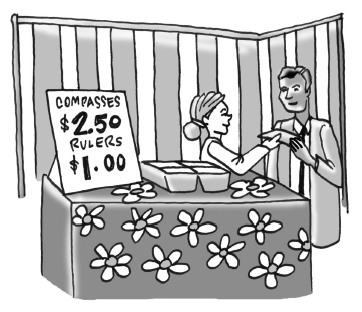### Home > CCA > Chapter Ch9 > Lesson 9.4.2 > Problem9-110

9-110.For the Spring Festival, the Math Club is selling rulers for $\1$ and compasses for $\2.50$.

1. While the club would like to sell as many items as they can to raise funds, they need to make at least $\15.00$ to break even. Write an inequality to represent this situation. Let $r=$ the number of rulers sold and $c=$ the number of compasses sold.

$r+\2.50c\ge\15.00$

2. School rules state that the club can sell a maximum of $25$ items for the festival. Write an inequality for this constraint (limitation).

$r+c\le25$

3. Graph the inequalities from parts (a) and (b) on the same set of axes so that compasses are represented on the $x$-axis and rulers are represented on the $y$-axis. Find the region of points that are solutions to each of them. Can this region fall below the $x$-axis or to the left of the $y$‑axis? Why or why not?

Test a point on one side of each line in order to find out what side must be shaded.

4. What do the points in the solution region represent?

The shaded region represents all of the points that satisfy both inequalities.

Use the blank expression lines in box 8 and 9 in the eTool below to graph the inequalities.
Click on the link at right for the full eTool version: CCA 9-110 HW eTool